Spitzer Documentation & Tools
IRS Instrument Handbook

### 2.10.3         Spectroscopic Sensitivity Correction for Extended Sources

The calculations and sensitivity curves shown above apply only to observations of point sources.  We provide a rough guide to the conversion from point- to extended-source sensitivities as follows.  Note that the approximations used here are only good to about 20% because of wavelength dependence in the conversion factors that varies from module to module.  The ESSC and ESSL are the 1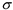staring extended source sensitivities per pixel for continuum and line, respectively.  They are: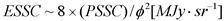Equation 2.8

and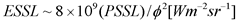Equation 2.9

where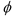is the linear edge size of a pixel projected onto the sky in arcseconds (Table 2.1, and different for each IRS module).  The values of PSSC (or its smoothed equivalent for a variety of AOT integration times) and PSSL can be read from the sensitivity curves above.

For example, the 1-sigma point source sensitivity (PSSC) of the SL module at 8 microns is 1.05 mJy in 6 seconds (from the sensitivity curve in Figure 2.15).  The pixel size for the SL module is 1.8 arcseconds.  So, the corresponding extended source sensitivity for SL is given by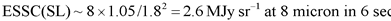Equation 2.10

Similar calculations can be carried out for the other IRS modules and exposure times.